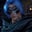Related Tags

java
communitycreator

# How to print alternate elements of an array in JavaGutha Vamsi Krishna

### Problem statement

You are given an array of size N. You need to print elements for the given array in an alternate order, starting from index 0.

### Example

Input:

• arr = {11,12,13,14}

Output:

• 11 13

#### Explanation

Since we need to start from index 0, we print 11, skip 12, and print 13.

### Solution

• Initialize the array arr with input numbers as 11, 12, 13, 14, 15.

• Find array length n, with length property on the array.

• Use the for loop to traverse the array.

• Start with index 0, go until the last element, and increment index by 2 every time to skip alternate elements.

• Print element.

When the loop ends, we will have printed all the alternate elements in the array.

### Code

class Solution {
public static void main( String args[] ) {
//initialize array
int[] arr = {11, 12, 13, 14, 15};

//array length
int n = arr.length;

// loop through the array and increment by 2
for(int i=0; i<n; i = i+2){
//print element
System.out.println(arr[i]);
}
}
}

### Time and space complexity

This solution takes $O(n)$ time complexity, as we traverse elements in the array once.

The solution then takes $O(1)$ space complexity, as we are not taking extra space to solve this problem aside from the input array.

RELATED TAGS

java
communitycreator

CONTRIBUTORGutha Vamsi Krishna
RELATED COURSES

View all Courses

Keep Exploring

Learn in-demand tech skills in half the time